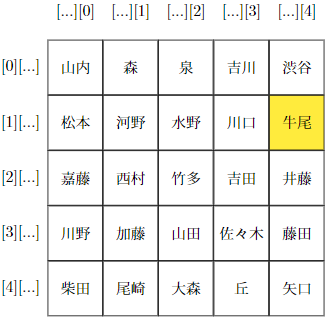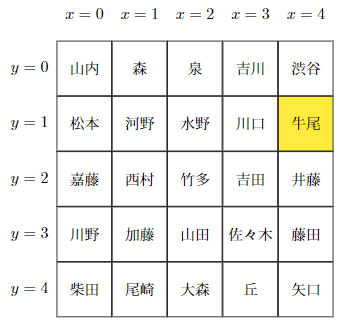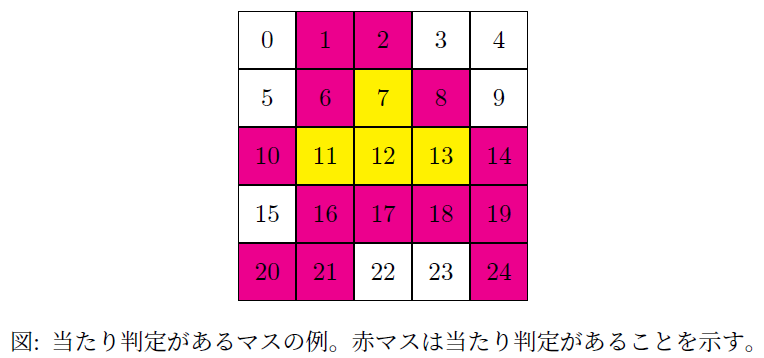# Introduction

I think that two-dimensional arrays are often used as a way to manage data with two-way indexes.

#### `Two-dimensional array example`

``````
String[][] names = {
{"Matsumoto", "Kono", "Mizuno", "Kawaguchi", "Ushio"},
{"Kato", "Nishimura", "Taketa", "Yoshida", "Ito"},
{"Shibata", "Ozaki", "Omori", "hill", "Yaguchi"}
};
System.out.println(names); //Ushio
``````I thought that if you manage with a two-way index, you can manage the data even if it is a one-dimensional array if you prepare a two-way index, so I decided to imitate the idea of a two-dimensional array with a one-dimensional array. [^ Experiment].

# Preparation

First, define two variables that indicate the width and height of the two-dimensional array. Hereafter, \$ width \$ will be referred to as ** array width **, and \$ height \$ will be referred to as ** array height **.

#### `python`

``````
int width = 5;
int height = 5;
``````

Then, prepare a one-dimensional version of the two-dimensional array mentioned earlier [^ Person class].

#### `python`

``````
String[] names = {
"Matsumoto", "Kono", "Mizuno", "Kawaguchi", "Ushio",
"Kato", "Nishimura", "Taketa", "Yoshida", "Ito",
"Shibata", "Ozaki", "Omori", "hill", "Yaguchi"
};
Iterator<String> nameTokens = Arrays.stream(names).iterator();
Person[] people = IntStream.range(0, width * height)
.mapToObj(i -> new Person(nameTokens.next()))
.toArray(Person[]::new);
``````Here is a diagram of the array. This array is an array with both ** array width ** and ** array height **. If the horizontal index of each row is \$ x \$ and the vertical index of each column is \$ y \$, then [^ x and y], for example, the index indicating "Ushio" is \$ x = 4, \ y = 1 . It becomes. Also, the horizontal index on the last element of each row is \$ x = width-1 \$, and the vertical index on the last element of each column is \$ y = height-1 \$\$.

# Practice

## Scanning elements

The element is scanned using the following code.

#### `Scan code`

``````
for (int i = 0, x = 0, y = 0; i < array.length; i++, x++) {
...
if (x == width - 1) {
x = -1;
y++;
}
}
``````

Scans all the elements of the array of interest (from index 0 to ʻarray.length`). This time we are defining a people array, so replace ʻarray.length` with` people.length`. The counter variables declared in the for statement are:

variable meaning
`i` Array index
`x` A value that indicates the horizontal index of the array
`y` A value that indicates the vertical index of the array

ʻIf (x == width --1) {...}`increments`y` if it is the last element of the line after processing the` ... `part of the scan code, and then Means to move to the line of. `x = -1` is for adjustment.

## Extracting and replacing elements

Using this, for example, in the `...` part of the scanning code

#### `python`

``````
if (y == 1 && x == 4) System.out.println(people[i]);
``````

If you define, you can output "Ushio".

#### `Extraction example`

``````
for (int i = 0, x = 0, y = 0; i < array.length; i++, x++) {
if (y == 1 && x == 4) System.out.println(people[i]); // Person [name=Ushio]
if (x == width - 1) {
x = -1;
y++;
}
}
``````

Also,

#### `python`

``````
if (y == 1 && x == 4) array[i] = new Person("Hoge");
``````

You can replace "Ushio" with "Hoge" by defining.

#### `Replacement example`

``````
for (int i = 0, x = 0, y = 0; i < array.length; i++, x++) {
if (y == 1 && x == 4) array[i] = new Person("Hoge");
if (x == width - 1) {
x = -1;
y++;
}
}
``````

I thought it would be a hassle to define it manually every time, so I modularized it [^ dirty].

Matrix class

#### `python`

``````
class Matrix<T, R> {
private T[] array;
public final int width;
public final int height;
public int i;
public int x;
public int y;
private Consumer<Matrix<T, R>> action;
private Function<Matrix<T, R>, R> function;

private Matrix(T[] array, int width, int height) {
this.array = array;
this.width = width;
this.height = height;
}

static <T, R> Matrix<T, R> of(T[] array, int width, int height) {
if (ObjectUtils.isEmpty(array))
throw new IllegalArgumentException("The array taken as the first argument is empty or null.");
if (array.length != width * height)
throw new IllegalArgumentException("The length of the array taken as the first argument and the value of "second argument x third argument" do not match.");
return new Matrix<>(array, width, height);
}

Matrix<T, R> setAction(Consumer<Matrix<T, R>> action) {
if (Objects.nonNull(function)) function = null;
this.action = action;
return this;
}

Matrix<T, R> setAction(Function<Matrix<T, R>, R> function) {
if (Objects.nonNull(action)) action = null;
this.function = function;
return this;
}

@SuppressWarnings("unchecked")
R get(int xIndex, int yIndex) {
if (isIllegalIndex(xIndex, yIndex)) return null;
return setAction(m -> {
if (m.y == yIndex && m.x == xIndex) return (R) array[m.i];
return null;
})
.run();
}

Matrix<T, R> put(T obj, int xIndex, int yIndex) {
if (isIllegalIndex(xIndex, yIndex)) return this;
setAction(m -> {
if (m.y == yIndex && m.x == xIndex) array[m.i] = obj;
})
.run();
return this;
}

T[] array() {
return array;
}

Matrix<T, R> insertShiftLeft(T obj, int xIndex, int yIndex) {
if (isIllegalIndex(xIndex, yIndex)) return this;
setAction(m -> {
if (m.i < width * yIndex + xIndex) array[m.i] = array[m.i + 1];
if (m.y == yIndex && m.x == xIndex) array[m.i] = obj;
})
.run();
return this;
}

Matrix<T, R> insertShiftRight(T obj, int xIndex, int yIndex) {
if (isIllegalIndex(xIndex, yIndex)) return this;
T[] cloneArray = array.clone();
setAction(m -> {
if (width * height - m.i - 1 > width * yIndex + xIndex)
array[width * height - m.i - 1] = cloneArray[width * height - m.i - 2];
if (m.y == yIndex && m.x == xIndex) array[m.i] = obj;
})
.run();
return this;
}

R run() {
if (Objects.isNull(action) && Objects.isNull(function))
throw new IllegalStateException("Define sequential processing.");
R result = null;
Supplier<R> l_action = null;
if (Objects.nonNull(action))
l_action = () -> {action.accept(this); return null;};
else if (Objects.nonNull(function))
l_action = () -> function.apply(this);
for (int i = 0, x = 0, y = 0; i < array.length; i++, x++) {
this.i = i;
this.x = x;
this.y = y;
result = l_action.get();
if (Objects.nonNull(result)) break;
if (x == width - 1) {
x = -1;
y++;
}
}
return result;
}

private boolean isIllegalIndex(int xIndex, int yIndex) {
if (xIndex < 0 || width  - 1 < xIndex) return true;
if (yIndex < 0 || height - 1 < yIndex) return true;
return false;
}
}
``````

I assumed both no return value and with return value. Specify the array type in the `T` part of`<T, R> of (...)`. For the `R` part, if there is a return value, specify the return type. If there is no return value, specify `java.lang.Void` and specify that there is no return value [^ null Void].

#### `Usage example (no return value)`

``````
Matrix.<Person, Void>of(people, width, height)
.setAction(m -> {
if (m.y == 1 && m.x == 4) System.out.println(people[m.i]); // Person [name=Ushio]
})
.run();
``````

Define the `...` part of the scan code with the setAction method before calling the run method. It uses a functional interface, replacing ʻi`, `x`, and` y`with`m.i`,` m.x`, and `m.y`, respectively. `m` does not have to be` m` because it is the argument name [^ m] of the lambda expression.

If there is a return value, it is a little complicated, but it uses a functional interface with `return` as follows. Define and use to return a non-null object when some condition is met. Also, if it does not match the condition, it will return null [^ null].

#### `Usage example (with return value)`

``````
String personName = Matrix.<Person, String>of(people, width, height)
.setAction(m -> {
if (m.y == 1 && m.x == 4) return people[m.i].toString();
return null;
})
.run();
System.out.println(personName); // Person [name=Ushio]
``````

These operations are implemented as get method and put method.

#### `python`

``````
Matrix<Person, Person> m = Matrix.of(people, width, height);
System.out.println(m.get(0, 3)); // Person [name=Kawano]
System.out.println(m.get(3, 2)); // Person [name=Yoshida]
System.out.println(m.get(4, 1)); // Person [name=Ushio]

m.put(new Person("Hoge"), 0, 3);
System.out.println(m.get(0, 3)); // Person [name=Hoge]
``````

## Insert and shift elements

Since it uses a one-dimensional array, you can insert an element by defining it as follows. Since the length of the array is fixed, the elements before "Yamada" are shifted one by one to the left, and the first element (Yamauchi) disappears.

#### `Insert a "hoge" between "Yamada" and "Sasaki" and shift to the left.`

``````
int xIndex = 2;
int yIndex = 3;
Matrix.<Person, Void>of(people, width, height)
.setAction(m -> {
if (m.i < width * yIndex + xIndex) array[m.i] = array[m.i + 1];
if (m.y == yIndex && m.x == xIndex) array[m.i] = new Person("Hoge");
})
.run();
``````

On the contrary, if you define as follows, the elements after "Yamada" will be shifted to the right one by one, and the last element (Yaguchi) will disappear. If you operate with only one array, the element shift when \$ x = 0 \$ is specified will not be as expected due to the problem of reference copy, so duplicate the array once and operate from that array to the original array. I am.

#### `Insert "Hoge" between "Kato" and "Yamada" and shift to the right.`

``````
int xIndex = 2;
int yIndex = 3;
Person[] cloneArray = people.clone();
Matrix.<Person, Void>of(people, width, height)
.setAction(m -> {
if (width * height - m.i - 1 > width * yIndex + xIndex)
array[width * height - m.i - 1] = cloneArray[width * height - m.i - 2];
if (m.y == yIndex && m.x == xIndex) array[m.i] = new Person("Hoge");
})
.run();
``````

These operations are implemented as insertShiftLeft method and insertShiftRight method.

#### `python`

``````
Matrix.<Person, Void>of(people, width, height)
.insertShiftLeft(new Person("Hoge"), 2, 4); // 「大森」と「丘」の間に「Hoge」を挿入し、左にシフトする。
``````

#### `python`

``````
Matrix.<Person, Void>of(people, width, height)
.insertShiftRight(new Person("Hoge"), 2, 4); // 「尾崎」と「大森」の間に「Hoge」を挿入し、右にシフトする。
``````

# Summary

Even with a one-dimensional array, we were able to manage data in a two-dimensional array. If you want to change the array width or array height later, you can handle it by simply changing the values of \$ width \$ and \$ height \$.

#### `python`

``````
int width = 7;
int height = 2;
String[] names = {
"Yamauchi", "Woods", "Izumi", "Yoshikawa", "Shibuya", "Matsumoto", "Kono",
"Kato", "Nishimura", "Taketa", "Yoshida", "Ito", "Kawano", "Kato"
};
Iterator<String> nameTokens = Arrays.stream(names).iterator();
Person[] people = ... //abridgement
Matrix<Person, Person> m = Matrix.of(people, width, height);
System.out.println(m.get(3, 1)); // Person [name=Yoshida]
``````

Alternatively, if you want to do it, you can define a method in the Matrix class that extracts one non-null element on the far left of each row, like this: By using the variables of the scanning code, you can output the elements on the right side, the upper side, and the lower side.

#### `python`

``````
@SuppressWarnings("unchecked")
public T[] getNonNullRightElements() {
T[] elements = (T[]) Array.newInstance(array.getClass().getComponentType(), height);
setAction(m -> {
if (Objects.nonNull(array[m.i])) elements[m.y] = array[m.i];
})
.run();
return ArrayUtils.removeAllOccurrences(elements, null);
}
``````

By applying this, it is also possible to pinpoint the squares with collision detection as shown in the following figure.# Postscript

## Modify the Matrix class

@ sdkei's comment pointed out the Matrix class. Thank you! Based on the improvement measures I received, I modified the Matrix class as follows.

Matrix class

#### `python`

``````
public final class Matrix<T, R> {
private final T[] array;
private final int width;
private final int height;
private MatrixRunner runner;
private MatrixSupplier<R> supplier;

private Matrix(T[] array, int width, int height) {
this.array = array;
this.width = width;
this.height = height;
}

public static <T, R> Matrix<T, R> of(T[] array, int width, int height) {
if (ObjectUtils.isEmpty(array))
throw new IllegalArgumentException("The array taken as the first argument is empty or null.");
if (array.length != width * height)
throw new IllegalArgumentException("The length of the array taken as the first argument and the value of "second argument x third argument" do not match.");
return new Matrix<>(array.clone(), width, height); //Defensive copy
}

public Matrix<T, R> setAction(MatrixRunner runner) {
if (Objects.nonNull(supplier)) supplier= null;
this.runner = runner;
return this;
}

public Matrix<T, R> setAction(MatrixSupplier<R> supplier) {
if (Objects.nonNull(runner)) runner= null;
this.supplier = supplier;
return this;
}

@SuppressWarnings("unchecked")
public R get(int xIndex, int yIndex) {
if (indexOutOfBounds(xIndex, yIndex)) return null;
return (R) array[width * yIndex + xIndex];
}

public Matrix<T, R> put(T obj, int xIndex, int yIndex) {
if (indexOutOfBounds(xIndex, yIndex)) return this;
array[width * yIndex + xIndex] = obj;
return this;
}

public T[] array() {
return array.clone(); //Defensive copy
}

public Matrix<T, R> insertShiftLeft(T obj, int xIndex, int yIndex) {
if (indexOutOfBounds(xIndex, yIndex)) return this;
setAction((i, x, y) -> {
if (i < width * yIndex + xIndex) array[i] = array[i + 1];
if (y == yIndex && x == xIndex) array[i] = obj;
})
.run();
return this;
}

public Matrix<T, R> insertShiftRight(T obj, int xIndex, int yIndex) {
if (indexOutOfBounds(xIndex, yIndex)) return this;
T[] cloneArray = array.clone();
setAction((i, x, y) -> {
if (width * height - i - 1 > width * yIndex + xIndex)
array[width * height - i - 1] = cloneArray[width * height - i - 2];
if (y == yIndex && x == xIndex) array[i] = obj;
})
.run();
return this;
}

public R run() {
if (Objects.isNull(runner) && Objects.isNull(supplier))
throw new IllegalStateException("Define sequential processing.");
R result = null;
MatrixSupplier<R> l_supplier = null;
if (Objects.nonNull(runner))
l_supplier = (i, x, y) -> { runner.run(i, x, y); return null; };
else if (Objects.nonNull(supplier))
l_supplier = (i, x, y) -> supplier.get(i, x, y);
for (int i = 0, x = 0, y = 0; i < array.length; i++, x++) {
result = l_supplier.get(i, x, y);
if (Objects.nonNull(result)) break;
if (x == width - 1) {
x = -1;
y++;
}
}
return result;
}

@FunctionalInterface
public interface MatrixRunner {
void run(int i, int x, int y);
}

@FunctionalInterface
public interface MatrixSupplier<R> {
R get(int i, int x, int y);
}

private boolean indexOutOfBounds(int xIndex, int yIndex) {
if (xIndex < 0 || width  - 1 < xIndex) return true;
if (yIndex < 0 || height - 1 < yIndex) return true;
return false;
}
}
``````

### Correction points

• Limitation of variability (`final class`, part of field` private final`, implementation of defensive copy) [^ immutable class].
• Weight reduction of get and put methods
• Introduction of MatrixRunner and MatrixSupplier interfaces. This eliminates the need to have a value as a `public` field to call the variables in the scan code.
• Modify other method names, make class methods `public`, etc.

By creating my own functional interface, I was able to make the encapsulation stronger. To be honest, I was impressed.

In addition, a runOnly method has been added to take advantage of the variability of the runner and supplier fields.

runOnly method

#### `python`

``````
public Matrix<T, R> runOnly() {
if (Objects.isNull(runner))
throw new IllegalStateException("Define sequential processing.");
for (int i = 0, x = 0, y = 0; i < array.length; i++, x++) {
runner.run(i, x, y);
if (x == width - 1) {
x = -1;
y++;
}
}
return this;
}
``````
Example of use

#### `python`

``````
@test
public void testRunOnly() {
List<String> people = new ArrayList<>();
matrix.setAction((i, x, y) -> {
if (x == 0 &&
(this.people[i].name.contains("Mountain") || this.people[i].name.contains("river"))
})
.runOnly()
.setAction((i, x, y) -> {
if (x == 3 && (this.people[i].name.contains("Kichi")))
I think that it is not very practical to process sequentially, but after performing one process with `runOnly ()`, once throw the Matrix object to another method or object, different actions within the other method or object After setting with the setAction method, if you use it by calling `runOnly ()` and performing different processing, I feel that the implementation of processing will be somewhat wider.